Home / Magnetism / Fundamental Laws of Magnetism

# Fundamental Laws of Magnetism

Want create site? Find Free WordPress Themes and plugins.

Today, a magnet can be defined as a material or substance that has the power to attract iron, steel, and other magnetic materials.

Through laboratory experiments, it was discovered that the greatest attractive force appeared at the ends of a magnet. These concentrations of magnetic force are called magnetic poles.

Each magnet has a north pole and a south pole. It was also discovered that many invisible lines of magnetic force existed between poles. Each line of force was an independent line. None of the lines cross or touch a bordering line.

Figure 1 shows the magnetic lines of force. This field picture was created by placing a bar magnet beneath a sheet of paper, and sprinkling it with fine iron filings.

Notice the pattern of lines existing between the poles. These lines of filings reflect the lines of force. Note the concentration of lines at each end of the magnet, or its poles. The lines of force are more concentrated at the poles.Figure 1. Magnetic lines of force can be “seen” by sprinkling iron filings across the lines of force.

Each magnetic line of force travels from the North Pole to the South Pole through space. The line returns to the North Pole through the magnet itself. These closed loops of the magnetic field can be described as magnetic circuits.

Compare the magnetic circuit to the electrical circuit. The magnetizing force can be compared to voltage, and the magnetic lines of force can be compared to current.

Further scientific investigation showed that the earth acts as one enormous magnet. The earth’s magnetic poles are close to the north and south geographic poles. Refer to Figure 2.

You can observe that magnetic north and the north geographic pole do not coincide. A compass would not necessarily point toward true north. This angle between true north and magnetic north is called the angle of declination or the angle of variation.Figure 2. The earth is a large magnet, surrounded by a magnetic field

There is, however, a line around the earth where the angle of declination is zero. When standing on this line, your compass would point to true north as well as magnetic north. At all other locations on the surface of the earth, the compass reading must be corrected to find true north.

## First and Second Laws of Magnetism

In Figure 3, two bar magnets have been hung in wire saddles and are free to turn. Notice that when the north (N) pole of one magnet is close to the south (S) pole of the other, an attractive force brings the two magnets together.

If the magnets are turned so that two N poles or two S poles are close to each other, there is a repulsive force between the two magnets. This demonstrates two of the laws of magnetism. These laws are stated:

1. Like poles repel each other.

2. Unlike poles attract each other.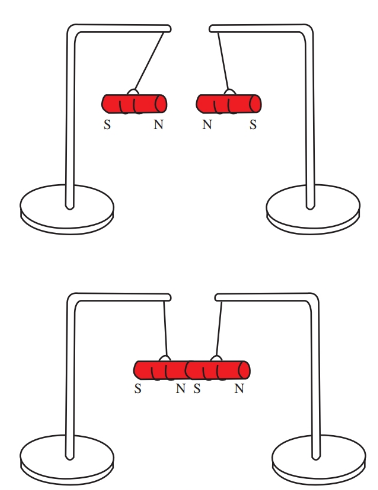Figure 3. These permanent magnets demonstrate the laws of magnetism.

Place the two magnets under a sheet of paper. Using the iron filings to detect the invisible fields, observe that in the attractive position (N and S together) the invisible field is very strong between the two poles, Figure 4 (top).

However, when the magnets are placed with like poles together, the repulsive force is shown by an absence of lines between the poles, Figure 4 (bottom).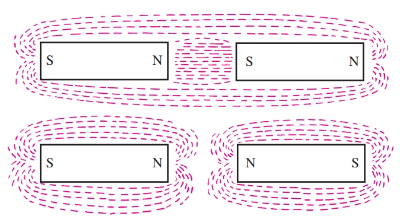Figure 4. These sketches show the magnetic fields of attracting and repelling magnets.

Caution:

Certain precautions should be observed during the handling and storage of permanent magnets. Magnetism is a result of molecular alignment. Any rough handling, such as the dropping or pounding of the magnets, can upset the molecular alignment and weaken the magnet.

## What causes a substance to become magnetized?

The molecules in an iron bar act as tiny magnets. If these tiny magnets are in a random order, Figure 5 (top), the bar does not act as a magnet. However, when these tiny magnets are arranged so that their north and south poles are in line, Figure 5 (bottom), the iron is magnetized. This can be demonstrated by breaking a piece of magnetized iron into several pieces. Each of the broken pieces acts as a separate magnet. Figure 6 shows a broken magnet. When the iron is demagnetized, these molecules are placed back in random positions.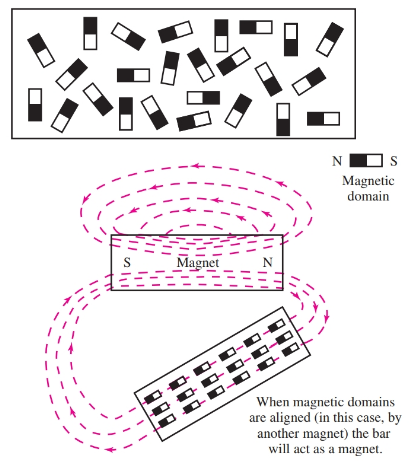Figure 5. Top—Molecules are not aligned, no magnetic force. Bottom—The molecules have been aligned by a nearby magnet. A magnet is created.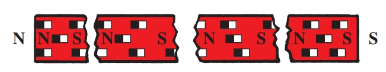Figure 6. A long magnet may be broken into several smaller magnets.

This molecular action is further demonstrated by the way a magnet is made. For example, take an unmagnetized iron bar. Rub it a few times in the same direction with a permanent magnet. A test (bring the bar near some iron filings) will show that the bar is now magnetized.

Rubbing the iron bar with the magnet lines up the molecules and causes the iron to become magnetized. Permanent magnets are made by placing the material to be magnetized in a very strong magnetic field.

Caution:

Bar magnets should be stored in pairs with north and south poles together to ensure their long life. A single horseshoe magnet may be preserved by placing a small piece of soft iron, called a keeper, across its poles.

Permanent magnets are made in a number of shapes and sizes, Figure 7. Flexible magnets may be used as a visual teaching aid. They are held in place on sheet iron by magnetism. Industry uses these boards for traffic control, production flow, temporary notices, and other announcements.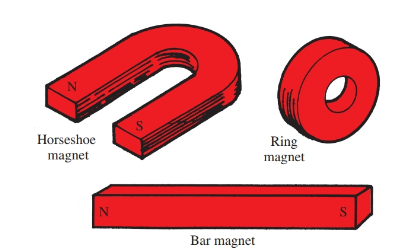Figure 7. Magnets are made in a number of styles, shapes, and sizes. Note that N and S poles of the ring magnet cannot be identified.

You will find similar (but smaller) flexible magnets on refrigerators in almost any home, Figure 8. These magnets are created by mixing steel filings in with the rubber. These flexible rubber/steel combinations are then placed in a magnetic field.Figure 8. Flexible magnets are made of a mix of steel and rubber.

## Magnetic Flux

The many invisible lines of magnetic force surrounding a magnet are called the magnetic flux.

If a magnet is strong, these lines of flux will be denser. So, the strength of a magnetic field can be determined by the field’s flux density, or the number of lines per square inch or per square centimeter. Flux density is expressed by the equation:

$B=\frac{\phi }{A}$

Where B equals flux density,$\phi$ (the Greek letter phi) equals the number of lines, and A equals the cross-sectional area.

The cross-sectional area can be measured in square inches or square centimeters. If the cross-sectional area is measured in square centimeters, then the flux density is given in the unit gauss. A gauss is the number of lines per square centimeter. The flux, B, is usually given in webers per square meter.

## Third Law of Magnetism

A simple experiment helps to demonstrate a third law of magnetism. Place one bar magnet on a table. Slowly slide one pole of a second bar magnet toward the opposite pole of the first bar magnet sitting on the table. When the two magnets get close enough together, the magnet on the table will be drawn to the second magnet. This demonstrates that:

The attractive force increases as the distance between the magnets decreases.

When the attractive force gains enough strength to overcome the force of friction holding the first magnet to the table, the first magnet slides toward the second. This magnetic force, either attractive or repelling, varies inversely with the square of the distance between the poles.

For example, if the distance between two magnets with like poles is increased to twice the distance, the repulsive force reduces to one-quarter of its former value. This valuable information explains the reason for accurately setting the gap in magnetic relays and switches. The term gap is the distance between the core of the electromagnet and its armature.

Did you find apk for android? You can find new Free Android Games and apps.

### About Ahmad FaizanMr. Ahmed Faizan Sheikh, M.Sc. (USA), Research Fellow (USA), a member of IEEE & CIGRE, is a Fulbright Alumnus and earned his Master’s Degree in Electrical and Power Engineering from Kansas State University, USA.